# Surface area + percentages - math problems

#### Number of problems found: 45

• Metal sheets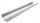How much metal sheet is needed to produce 8 gutters 4 m long and 12 cm in diameter? During production, joints calculate at 3% of total consumption.
• The surface areaHow much percent will the surface area of a 4x5x8 cm block increase if the length of the shortest edge is increased by 2 cm?
• The ballThe ball has a radius of 2m. What percentage of the surface and volume is another sphere whose radius is 20% larger?
• Gutter pipe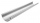How many m² of sheet metal is required to produce a 12 m long and 18 cm wide gutter, if 7% bend is required?The radius of the sphere we reduce by 1/3 of the original radius. How much percent does the volume and surface of the sphere change?
• The observatoryThe dome of the hemisphere-shaped observatory is 5.4 meters high. How many square meters of sheet metal needs to be covered to cover it, and 15 percent must be added to the minimum amount due to joints and waste?
• Tropical, mild and arctic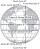How many percent of the Earth's surface lies in the tropical, mild, and arctic range? The border between the ranges is the parallel 23°27' and 66°33'.Alaska is the largest state in the United States and has a surface area of approximately 588,000 square miles. Montana has a surface area that is approximately 25% of the surface area of Alaska. What is the approximate surface area of Montana?
• Water tankA 288 hectoliter of water was poured into the tank with dimensions 12 m and 6 m bottom and 2 m depth. What part of the volume of the tank water occupied? Calculate the surface of tank wetted with water.
• Cube surface areaThe surface of the cube was originally 216 centimeters square. The surface of the cube has shrunk from 216 to 54 centimeters sq. Calculate how much percent the edge of the cube has decreased.
• Surface of wallFind by what percentage the surface of the cube will decrease if we reduce the surface of each of its walls by 12%.
• Surface area 2Calculate how many % reduce the surface area of the cube is we reduced length of each edge by 10%.
• Metal box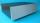How much metal we need for the production of box with dimensions 5 dm, 30 cm and a height of 1 m? Add 12% of the waste and folds.
• Earth's surface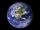The greater part of the earth's surface (r = 6371 km) is covered by oceans; their area is approximately 71% of the Earth's surface. What is the approximate area of the land?
• Cube zoomHow many percents do we increase the volume and surface of the cube if we magnify its edge by 38 %?
• GutterHow much metal is needed for production 60 pieces of gutter pipes with the diameter 17 cm and length of 6 m? The plate bends add 6% of the material.
• Cube wallThe surface of the first cube wall is 64 m2. The second cube area is 40% of the surface of the first cube. Determine the length of the edge of the second cube (x).
• Cardboard boxWe want to make a cardboard box shaped quadrangular prism with rhombic base. Rhombus has a side of 5 cm and 8 cm one diagonal long. The height of the box to be 12 cm. The box will be open at the top. How many square centimeters cardboard we need, if we ca
• Church roof 2The roof has the shape of a rotating cone shell with a base diameter of 6 m and a height of 2.5 m. How many monez (CZK) will cost the roof cover sheet if 1 m2 of metal sheet costs 152 CZK and if you need 15% extra for joints, overlays and waste?
• Iglu - cone tentThe cone-shaped tent is 3 m high, the diameter of its base is 3.2 m. a) The tent is made of two layers of material. How many m2 of fabric is needed for production (including flooring) if 20% needs to be added to the minimum amount due to cutting waste? b)

Do you have an exciting math question or word problem that you can't solve? Ask a question or post a math problem, and we can try to solve it.

We will send a solution to your e-mail address. Solved examples are also published here. Please enter the e-mail correctly and check whether you don't have a full mailbox.

Our percentage calculator will help you quickly calculate various typical tasks with percentages. Examples for the calculation of the surface area of ​​the solid object . Percentages Problems.